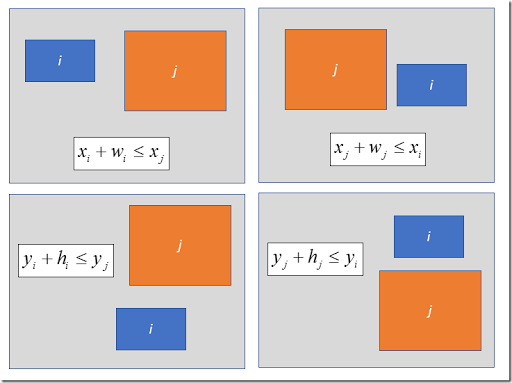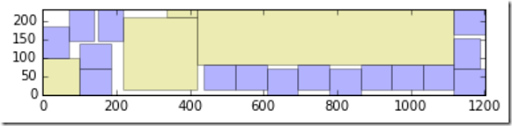The question of how we can formulate constraints that enforce rectangles not to overlap comes up regularly (1). There are basically four cases to consider when considering two rectangles $$i$$ and $$j$$:In the picture above, we indicate by $$(x_i,y_i)$$ the left-lower corner of a rectangle (these are typically decision variables). The height and the width are $$h_i$$ and $$w_i$$ (these are typically constants). From the above, we can formulate the “no-overlap”  constraints as:

 \bbox[lightcyan,10px,border:3px solid darkblue] {\begin{align}&x_i+w_i \le x_j & \text{or} \\&x_j+w_j \le x_i & \text{or} \\&y_i+h_i \le y_j & \text{or} \\&y_j+h_j \le y_i \end{align} }

The “or” condition can be modeled with the help of binary variables $$\delta$$ and big-M constraints:

 \bbox[lightcyan,10px,border:3px solid darkblue] {\begin{align}&x_i+w_i \le x_j + M_1 \delta_{i,j,1}\\&x_j+w_j \le x_i + M_2 \delta_{i,j,2}\\&y_i+h_i \le y_j + M_3 \delta_{i,j,3}\\&y_j+h_j \le y_i+ M_4 \delta_{i,j,4}\\&\sum_k \delta_{i,j,k} \le 3\\& \delta_{i,j,k} \in \{0,1\} \end{align} }

The binary variables make sure at least one of the constraints is active (not relaxed).

If we have multiple rectangles we need to compare all combinations, i.e. we generate these constraints for  $$\forall i,j$$. Except: we can not compare a rectangle with itself. A first approach would be to generate the above constraints for all $$i\ne j$$. This is correct, but we can apply an optimization. Note that we actually compare things twice: if rectangles $$i$$ and $$j$$ are non-overlapping then we don’t need to check the pair of rectangles $$j$$ and $$i$$. That means we only need constraints and variables $$\delta_{i,j,k}$$ for $$i<j$$.

It is important to make constants $$M_k$$ as small as possible. In general we have a good idea about good values for these $$M_{k}$$’s. E.g. consider the case where we need to pack boxes in a container (2):If the container has length and width $$H$$ and $$W$$ then we can easily see: $$M_1=M_2=W$$ and $$M_3=M_4=H$$.

##### References
1. How to write this constraint in LPSolve?, https://stackoverflow.com/questions/44858353/how-to-write-this-constraint-in-lp-solver-logical-and-and-doesnot-exist
2. Filling up a container with boxes, http://yetanothermathprogrammingconsultant.blogspot.com/2016/06/filling-up-container-with-boxes.html